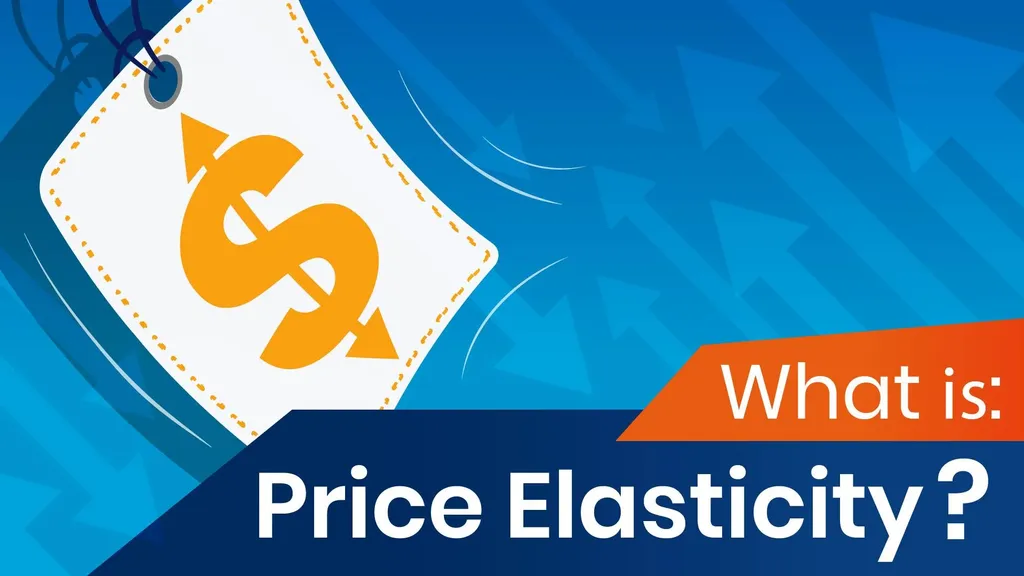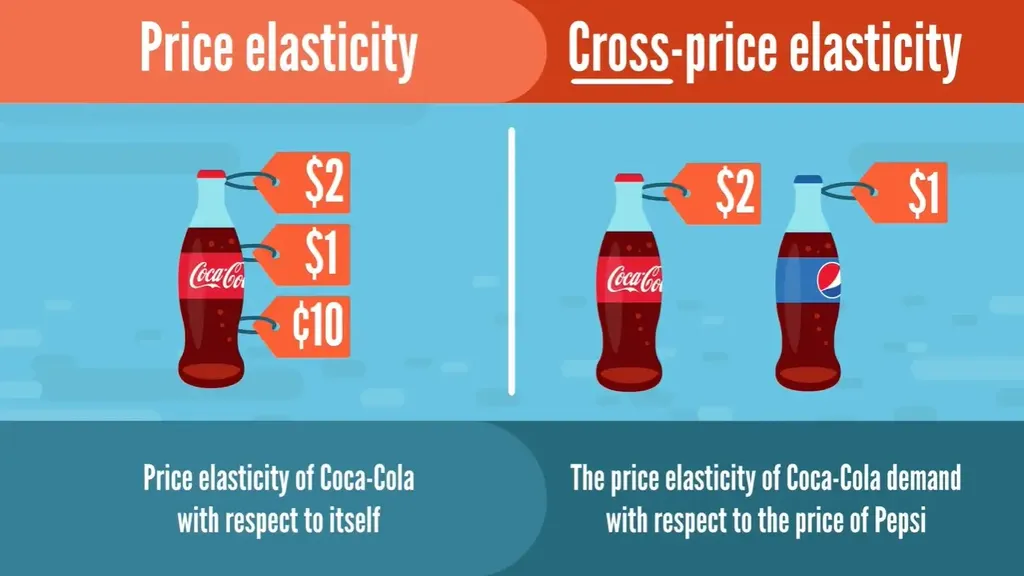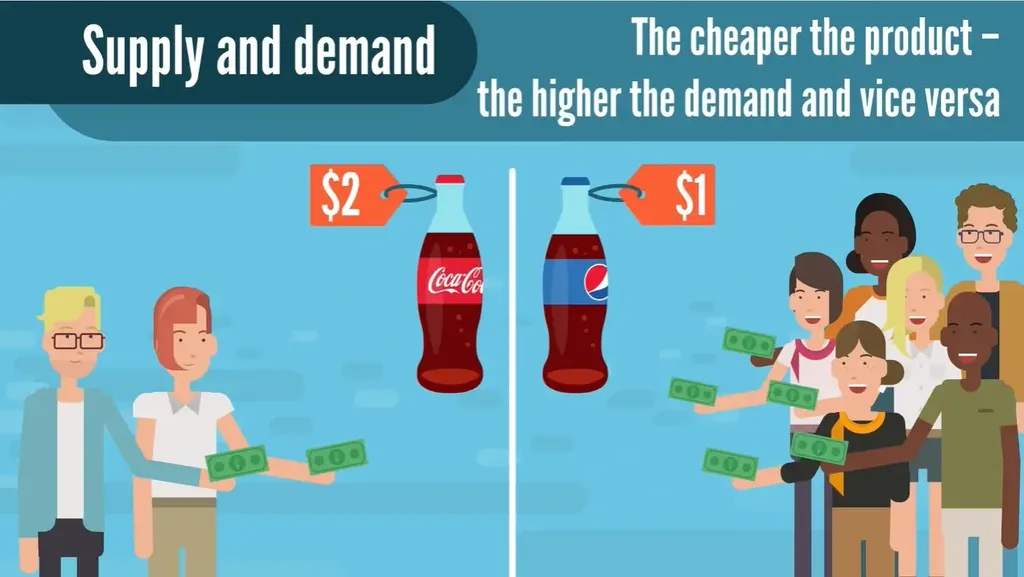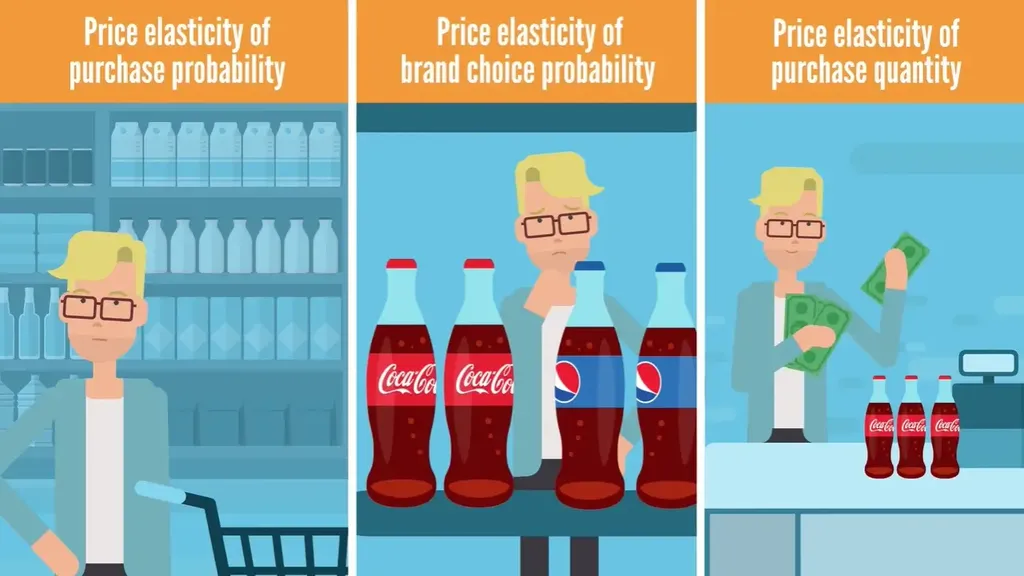# What Is Price Elasticity?Even if you’re no expert in economics, you’ll notice how prices in stores fluctuate. Sometimes it seems like there’s no rhyme or reason to them, doesn’t it? Well, we promise there’s a perfectly good explanation and it lies in one of the most important economic and marketing concepts – price elasticity.

In this article, we’ll introduce you to the definition of elasticity and provide examples. You’ll see how marketers apply it to track customer behavior and obtain meaningful insights. In addition, you will learn how this concept affects economic outcomes and how you can use them to your advantage.

## What Is Price Elasticity?In the broadest terms possible, price elasticity measures how purchasing behavior changes when the price changes.

For instance, let’s assume a bottle of Coca-Cola regularly costs \$1. If the price increases to \$2, many people would stop buying the brand, as it would be too expensive. On the other hand, if the price decreases to 10¢, more people are going to start purchasing this brand.

The measure we use to quantify this phenomenon is called own-price elasticity, price elasticity of demand, or simply price elasticity. In this case, because we’re calculating the elasticity of Coca-Cola with respect to itself, we call it price elasticity of Coca-Cola demand.

Now, as you can imagine, if a Pepsi also costs \$1 and Coca-Cola cuts its products’ price to 10¢, then most of the people that used to buy Pepsi would immediately switch to Coca-Cola. Meanwhile, if Coca-Cola prices increase to \$2, then people will likely stop buying from them and go with Pepsi instead. That is to say that there seems to be another important phenomenon - price elasticity of Coca-Cola demand with respect to the price of Pepsi, or the so-called cross-price elasticity.

In order to avoid any confusion, when we’ve got 2 products, we must say the whole name – price elasticity of Coca-Cola demand with respect to the price of Pepsi.

## What Is Price Elasticity of Supply and Demand?Price elasticity stems from the basic economic law of supply and demand:

• The cheaper the product – the higher the demand.
• The more expensive the product – the lower the demand.

It is extremely important for businesses, because of this sweet spot that maximizes revenue:

$Revenue = Price \times Units~sold$

Now, we can use elasticity to find the point at which the equation is optimal.

In mathematical terms, price elasticity (E) is the percentage change of an economic outcome of interest (usually the number of units sold) in response to a 1% change in the respective price:

$E = \frac {\%~Change~in~economic~outcome~of~interest~(Units~sold)}{1\%~Change~in~price}$

Let us denote the economic outcome of interest with Y and price with P. Then, the price elasticity of Y must reflect the percentage change in Y in response to a 1% change in the P:

$E = \frac {Y\%}{P\%}$

We can obtain that by taking the percentage change of Y and dividing it by the percentage change in P:

$E = \frac {\frac{\Delta Y}{Y}}{\frac{\Delta P}{P}}$

Well, the percentage change of Y is the difference between its present and past value, divided by the past value. Similarly, the percentage change of P is the difference between the present and past price, divided by the past price:

$E = \frac {\frac{Y_t-Y_{t-1}}{Y_{t-1}}}{\frac{P_t-P_{t-1}}{P_{t-1}}}$

## Price Elasticity: Economic OutcomesCustomer analytics concerns itself with estimating the following economic outcomes of interest:

1. Purchase probability – Will a customer buy a product from a particular product category when they enter the shop?
2. Brand choice probability – Which brand is the customer going to choose?
3. Purchase quantity – How many units is the customer going to purchase?

Naturally, we would be interested in the price elasticities of each of these economic outcomes, so we will look at all three.

### Price Elasticity of Purchase Probability

There may be many different brands with differing prices from the same product category on the market – for instance, the different brands of beer.

Suppose we can calculate an aggregate price for the whole category. The law of demand says that the greater the price, the lower the quantity that customers want to buy. So, if the aggregate price increases, the probability of purchasing a beer would decrease. Calculating price elasticity will show us how exactly much.

### Price Elasticity of Brand Choice

This is the most interesting and most important outcome for marketers.

If you are working for Oreo, you’d be interested in the Oreo brand – not in the aggregate biscuits sales across the board. That’s why a marketer devotes his entire efforts to influencing customers to choose namely their brand over their competitors.

Similar to purchase probability, we can assume that if the price of a product from a given brand increases, the brand choice probability for it decreases. Again, calculating price elasticity for a brand with respect to the price of its product would show us exactly how much.

Accordingly, if another brand increases its unit price, the brand choice probability would also increase. This is precisely the Coca-Cola vs. Pepsi example we started with. Such elasticity calculations will show us how much the brand choice probability would increase with a 1% increase in a competing brand’s prices.

### Price Elasticity of Purchase Quantity

As you might expect from following the law of demand – the greater the unit price of a product, the lower the quantity purchased.

For a car, the difference may be from 1 to 0. For example, if we find the price of a Tesla is acceptable, then we will buy 1 unit. If it is not – we won’t buy any. Alternatively, if we are considering avocados, for instance, we may decide to buy 0, 1, or even 10 units at once, depending on the price. Calculating the price elasticity will show us exactly how the purchase quantities move with the price change.

## Price Elasticity: Next Steps

While it might seem chaotic from an outside perspective, there’s a finely measured process behind product pricing. Elasticity is an important marketing that requires some in-depth knowledge about customer analytics and marketing.

To calculate price elasticity, you could use many statistical software packages, such as SPSS, SAS, or R. We recommend you use Python as it’s a widely used statistical computing environment and is possibly the most popular choice amongst data scientists. Try our Customer Analytics in Python course to gain even more insight into the world of data and customer analytics.

You can learn or upgrade your Python skills and work on your data science portfolio with the help of our 365 Data Science program. Starting from the very basics all the way to advanced specialization, you will learn by doing with a myriad of practical exercises and real-world business cases. If you want to see how the training works, start with our free lessons by signing up below.

Learn data science with industry expertsStart your career